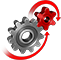# WolframSystemModeler

## Binary Counter

Counters are used in a variety of digital applications as a way of counting events. In this example, we have constructed a simple 4-bit asynchronous up-counter using the Digital library, which is a part of the Modelica Standard Library.

To run this example, you'll need

### Counter Model

Counters can be used for a huge array of applications. They can, for example, be used to count pulses from a sensor attached to a wheel to count the number of revolutions, which in turn can be used to calculate the speed of the wheel. Counters also can be used as digital clocks for different purposes. Another typical use of a digital counter is in central processing units (CPUs), where a certain kind of counter (program counters, or PCs) is used as a way for the CPU to walk through program instructions, one by one, from a memory. The model in this example consists of a 4-bit asynchronous up-counter that is fed with a clock pulse of 1 Hz. Below, you can see a diagram of the model.
Diagram view of the model. The counter in the diagram is fed by a clock pulse and an enable signal that tells the counter when to count and when to pause.
Flip-flops or latches are used as basic components in digital circuitry and work as a kind of memory that stores the state of one bit. By using multiple flip-flops, it is possible to construct digital state machines. A binary counter is basically a state machine that just cycles through its states for each cycle of a clock signal. The JK flip-flop is considered to be the most universal flip-flop design and can be used as different kinds of flip-flops just by adjusting how the input to the J and K terminals is done. In this example, the flip-flops are used with a toggling function, which means that the output is changed for each completed clock cycle. This is accomplished by feeding ones into both the J and K pins of the flip-flops. By putting only zeros on all the J and K terminals, the output will never change, regardless of the input. This makes it suitable to connect all the J and K terminals as an enable signal for the circuit.
The counter in this example is a 4-bit asynchronous counter based on JK flip-flops. The flip-flops are connected with both their J and K terminals to the enable pin, putting them in "toggle mode". The flip-flop to the left, producing the Q0 signal, will change its output state for each falling edge of the clock signal, for example, a CPU clock. Since the output toggles for each falling edge of the clock, the clock toggles twice for each toggle of the output.
This diagram from a simulation shows how the logic levels of the four bits change over time. The enable signal goes from 0 to 1 after one second.

Use Drag and Drop to Quickly Produce Results!

Design and simulate essential digital circuits efficiently by making use of SystemModeler’s drag-and-drop functionality.

## Wolfram System Modeler

Questions? Comments? Contact a Wolfram expert »